# CBSE Class 12 Chemistry Solid State Important Questions and Answers

1. Define unit cell.
A basic repeating structural unit of a crystalline solid is called a unit cell.

2. Give any three characteristics of ionic crystals.
• Ionic solids have high melting points.
• They do not conduct electricity in solid state.
• They conduct electricity in molten state (or) when dissolved in water.
• They are hard and brittle.

3. Differentiate crystalline solids and amorphous solids.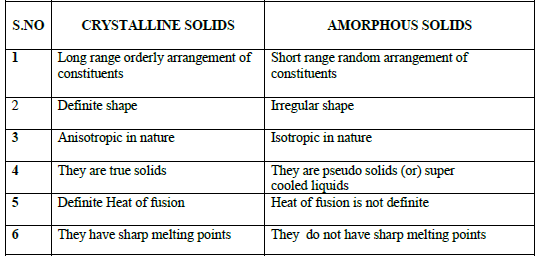4. Classify the following solids
a. P4
b. Brass
c. diamond
d. NaCl
e. Iodine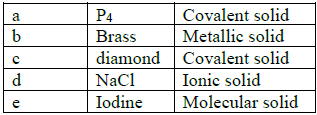5. Explain briefly seven types of unit cell.
There are seven primitive crystal systems. They differ in the arrangement of their crystallographic axes and angles.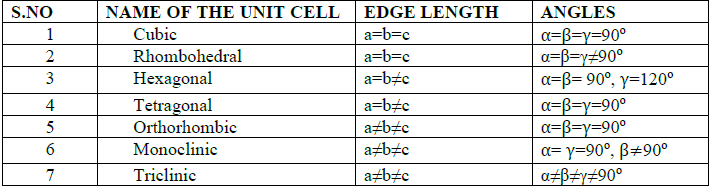6. Distinguish between hexagonal close packing and cubic close packing.7. Distinguish tetrahedral and octahedral voids.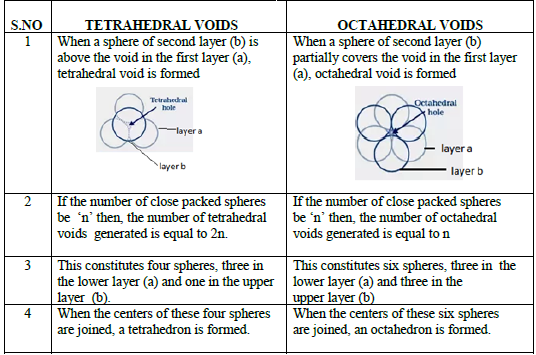8. What are point defects?

Point defects are the deviations from ideal arrangement that occurs at some points or atoms in a crystalline substance.

9. Explain Schottky defect.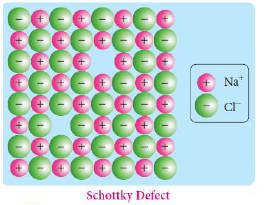• Schottky defect arises due to the missing of equal number of cations and anions from the crystal lattice .Hence stoichiometry of the crystal is not changed.
• Ionic solids in which the cation and anion are of almost of similar size show schottky defect.
Example: NaCl.
• Presence of large number of schottky defects in a crystal lowers its density.

10. Write short note on metal excess and metal deficiency defect with an example.(or) Write a short note on non-stoichiometric defects.

Metal excess defect: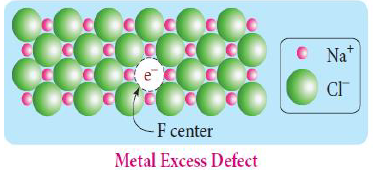• Metal excess defect arises due to the presence of more number of metal ions than the anions. Ex: NaCl, KCl
• The electrical neutrality of the crystal is maintained by the presence of anionic vacancies equal to the excess metal ions or by the presence of extra cation and electron present in interstitial position .
• The anionic vacancies, which are occupied by unpaired electrons are called F centers.

Metal deficiency defect: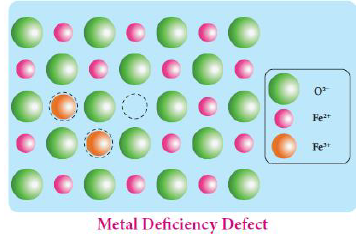• Metal deficiency defect arises due to the presence of less number of cations than the anions.
• This defect is observed in a crystal in which the cations have variable oxidation state. For example, in FeO crystal, some of the Fe2+ ions are missing from the crystal lattice. To maintain the electrical neutrality, twice the number of other Fe2+ oxidized to Fe3+ ions.
• In such cases, overall number of Fe2+ and Fe3+ ions is less than the O2- ions.

11. Calculate the number of atoms in an fcc unit cell.12. Explain AAAA and ABABA and ABCABC type of three dimensional packing with the help of neat diagram.

i. AAAA three dimensional packing:• It occurs in simple cubic arrangement.
• This type of three dimensional packing arrangements can be obtained by repeating the AAAA type two dimensional arrangements in three dimensions.
• Spheres in one layer sitting directly on the top of those in the previous layer so that all layers are identical.
• All spheres of different layers of crystal are perfectly aligned horizontally and also vertically.
• In simple cubic packing , each sphere is in contact with 6 neighbouring spheres- Four in its own layer, one above and one below and hence the coordination number is 6.

ii.  ABABA three dimensional packing: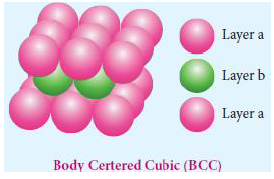• It occurs in body centeredcubic arrangement.
• In this arrangement, the spheres in the first layer(a) are slightly separated and the second layer (b) is formed by arranging the spheres in the depressions between the spheres in first layer (a) .
• The third layer is a repeat of the first.
• This pattern ABABAB is repeated throughout the crystal.
• In this arrangement, each sphere is in contact with 8 neighbouring spheres- Four in the above layer and four in the below layer and hence the coordination number is 8.

iii.   ABCABC three dimensional packing: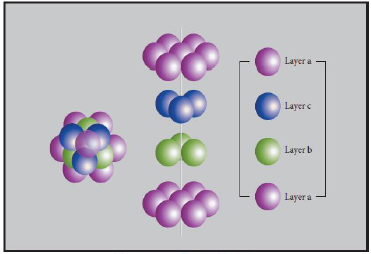• The first layer (a) is formed by arranging the spheres as in the case of two dimensional ABAB arrangements.
• The second layer (b) is formed by placing the spheres in the depressions of the first layer.
• A tetrahedral void(x) and octahedral voids(y) are formed in the first layer.
• The third layer is placed over the second layer in such a way that all the spheres of the third layer fit in octahedral voids.
• This arrangement of the third layer is different from other two layers (a) and (b) . Hence, the third layer is designated (c).
• If the stacking of layers is continued in abcabc...pattern, then the arrangement is called cubic close packed (ccp) structure.
• In ccp arrangements, the coordination number of each sphere is 12- six neighbouring spheres in its own layer, three spheres in the layer above and three sphere in the layer below.

13. Why ionic crystals are hard and brittle?
• Ionic crystal are hard due to strong electrostatic force of attraction between cations and anions.
• They are brittle because ionic bonds are non directional.

14. Calculate the percentage efficiency of packing in case of body centered cubic crystal.
In bcc, the spheres are touching along the leading diagonal of the cube as shown in the fig.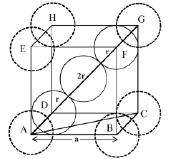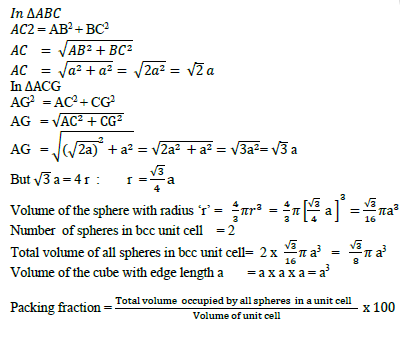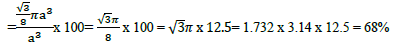15. What is the two dimensional coordination number of a molecule in square close packed layer?

The two dimensional coordination number of a molecule in square close packed layer is 4. In this arrangement each sphere is in contact with four of its neighbours.

16. Experiment shows that Nickel oxide has the formula Ni0.96 O1.00 . What fractionof Nickel exists as of Ni2+ and Ni3+ ions?
Number of Ni2+ ion = x
Number ofNi3+ ion = y
x + y = 0.96
x = 0.96 –y
The charge on Ni2+ ion and charge on Ni3+ ion are balanced by the charge on O2- ion.
Therefore,
2x + 3y = 2
2(0.96 – y) + 3y = 2
1.92 – 2y + 3y = 2
1.92+ y = 2
y = 2 – 1.92 = 0.08
x = 0.96– 0.08= 0.88
Percentage of Ni2+ inNi0.96 O1.00 = 0.88 x 100 / 0.96 =91.67 %
Percentage of Ni3+ inNi0.96 O1.00 = 0.08 x 100 / 0.96 =8.33 %

17. What is meant by the term “coordination number”? What is the coordination number of atoms in a bcc structure?

The neighbouring atoms surrounded by each atom is called coordination number. In the bodycentre, each atom is surrounded by eight nearest neighbours and coordination number is 8.

18. An element has bcc structure with a cell edge of 288 pm.The density of the element is 7.2 gcm-3. How many atoms are present in 208g of the element.
Given:
Edge of bcc (a)= 288pm = 2,88 x 10-8 cm (since density is given as gcm-3, the edge length should be converted to cm). For bcc, Z = 2
Density = 7.2 gcm-3
Mass of element = 208g19. Aluminium crystallizes in a cubic close packed structure. Its metallic radius is 125pm.Calculate the edge length of unit cell.
Given: r = 125pm.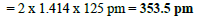20. If NaCl is doped with 10-2 mol percentage of strontium chloride, what is the concentration of cation vacancy?

100 moles of NaCl is dopped with 10-2 moles of SrCl2
Therefore 1 mole of NaCl is dopped with 10-2 / 100 = 10-4 moles of SrCl2
Each Sr2+ ion will create 1 cation vacancy in NaCl.
Numberof cationic vacancy produced by 10-4 mol Sr2+ ion = 6.023 x 1023 x 10-4
= 6.023 x 1019
Number of cation vacancies produced by SrCl2 = 6.023 × 1019 per mol

21. KF crystallizes in fcc structure like sodium chloride. calculate the distance between K+ and F in KF.( given : density of KF is 2.48 g cm−3)
Molar mass of KF = 39.1 + 19 = 58.1g/mol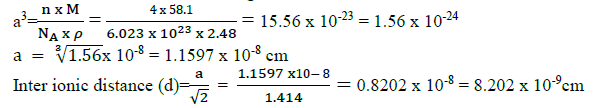22. An atom crystallizes in fcc crystal lattice and has a density of 10 gcm-3
with unit cell edge length of 100pm. Calculate the number of atoms present in 1 g of crystal.
Given:
Density =10 gcm-3:  a = 100 pm = 100 x 10 -10cm :  Mass = 1g
Number of atoms in fcc unit cell = 423. Atoms X and Y form bcc crystalline structure. Atom X is present at the corners of the cube and Y is at the centre of the cube. What is the formula of the compound?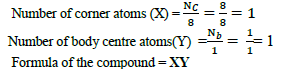24. Sodium metal crystallizes in bcc structure with the edge length of the unit cell 4.3 ×10−8cm. Calculate the radius of sodium atom.
Given: a = 4.3 ×10−8cm.
For bcc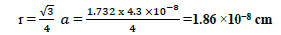25. Explain Frenkel defect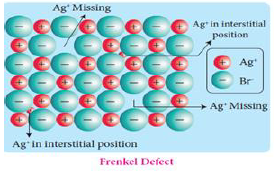• Frenkel defect arises due to the dislocation of ions from its crystal lattice.
• The ion which is missing from the lattice point occupies an interstitial position.
• This defect occurs when cation and anion differ in size.
• Unlike Schottky defect, this defect does not affect the density of the crystal.Ex: AgBr

II-EVALUATE YOURSELF:

1. An element has a face centered cubic unit cell with a length of 352.4 pm along an edge. The density of the element is 8.9 gcm-3. How many atoms are present in 100 g of an element?
Given: Density =8.9 gcm-3:  a = 352.4 pm = 3.524 x 10 -8cm , Mass = 100g , Z = 4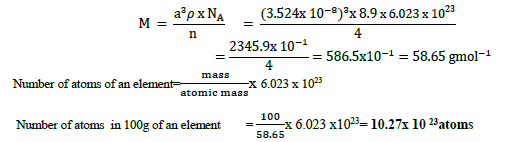2. Determine the density of CsCl which crystallizes in a bcc type structure with an edge length 412.1 pm.
Given: M=133 + 35.5 =168.5 gmol-1, a= 412.1 pm= 4.121 x 10-8 cm , Z=1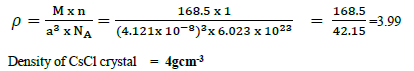3. A face centered cubic solid of an element (atomic mass 60) has a cube edge of 4A0. Calculate its density.

Given: M=60 gmol-1, a = 4 A= 4 x 10-8 cm , Z=4III-TEXTBOOK EXAMPLES:

1. Barium has a body centered cubic unit cell with a length of 508pm along an edge. What is the density of barium in g cm-3?
Given: M=137.3 gmol-1, a= 508pm = 5.08x 10-8cm , Z=21. How solids are classified?
Solids are classified into two types
(i) Crystalline solids
(ii) Amorphous solids..Ex: glass, rubber

Crystalline solids are further classified depending upon nature of their constitutents.
(a) Ioniccrystals. Ex: NaCl, KCl
(b) Covalent crystals Ex: Diamond, SiO2
(c) Molecular crystals. Ex: Naphthalene, Anthracene, Glucose
(d) Metallic crystals. Ex: Na,Mg,Au,Ag
(e) Atomic solids. Ex: Frozen elements of group 18

2. Explain isotropy and anisotropy ?
• Isotropy means having identical values of physical properties (refractive index, electrical conductance) in all directions. Ex-amorphous solids.
• Anisotropy means having different values of physical properties when measured along different directions. Ex- crystalline solids

3. Give a note on covalent solids ?
• In covalent solids, the constituents (atoms) are bound together in a three dimensional network entirely by covalent bonds
• Examples: Diamond, silicon carbide etc.
• Such covalent network crystals are very hard, and have high melting point.
• They are usually poor thermal and electrical conductors

4. What are molecular crystals? How are they classified?
• In molecular solids, the constituents are neutral molecules.
• They are held together by weak Vanderwaals forces.
• Generally, molecular solids are soft and they do not conduct electricity
• Molecular solids are further classified into three types.

5. Explain metallic solids.
• In metallic solids, the lattice points are occupied by positive metal ions and a cloud of electrons pervades the space.
• They are hard, and have high melting point.
• Metallic solids possess excellent electrical and thermal conductivity. They possess bright lustre.
• Examples: Metals and metal alloys belong to this type of solids, for example Cu, Fe, Zn, Ag ,Au, Cu- Zn etc.

6. Name the parameters that characterize a unit cell
A unit cell is characterised by the three edge lengths or lattice constants a ,b and c and the angle between the edges α, β and γ.

7. What are primitive and non-primitive unit cell?
A unit cell that contains only one lattice point is called a primitive unit cell,
A unit cell that contains additional lattice points, either on a face or within the unit cellis called anon- primitive unit cells.

8. Sketch i.sc ii.bcc iii.fcc&calculate its numberof atoms per unit cell9. How inter planar distance are calculated in unit cell?
The inter planar distance (d) between two successive planes of atoms can be calculated using the following equation form the X-Ray diffraction data
2dsin θ= n λ
The above equation is known as Bragg’s equation, where
λ-wavelength of X-ray used for diffraction. θ-angle of diffraction n – order of refraction
By knowing the values of λ, θ and n we can calculate the value of d.
d = nλ/2dsin θ

10. Explain the relationship between atomic radius and edge length of fcc unit cell and calculate its packing efficiency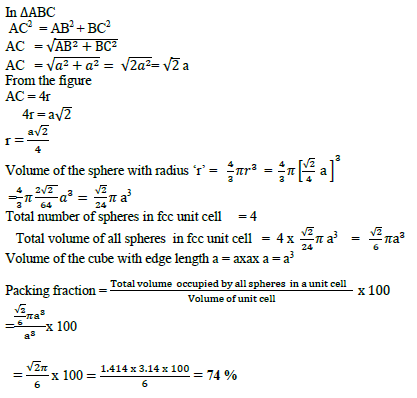11. Calculate the packing efficiency of polonium.
Polonium crystallizes in simple cubic pattern. So let us consider simple cubic system.
Let us consider a cube with an edge length ‘a’ as shown in fig.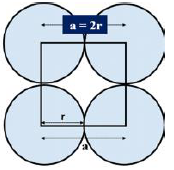Volume of the cube with edge length a is = a x a x a = a3
Let ‘r’ is the radius of the sphere.
From the figure, a=2r, r=a/212. Radius ratio of an ionic solid is found to be 0.415. Where the cations are occupied?
Cations occupies the octahedral voids.
{Hint: radius ratio <0.4 – tetrahedral voids
radius ratio >0.4 – octahedral voids}

13. Write the possible octahedral voids and tetrahedral voids exist per atom in a crystal.
Number of tetrahedral voids = 2
Number of octahedral voids = 1

14. How electrical neutrality is maintained in stoichiometric ionic crystals?
In stoichiometric ionic crystals, a vacancy of one ion must always be associated with either by the absence of another oppositely charged ion (or) the presence of same charged ion in the interstitial position so as to maintain the electrical neutrality.

15. Explain how vacancies are introduced in an ionic solid when a cation of higher valence is added as impurity in it. (Impurity defect)
• Due to the presence of impurity, ions in ionic solids causes vacancies in the crystal lattice of the host.
• For example, addition of CdCl2 to silver chloride yields solid solutions where the divalent cation Cd 2+ occupies the position of Ag+.
• This disturbs the electrical neutrality of the crystal.
• In order to maintain the same, proportional number of Ag+ ions leaves the lattice This produces a cation vacancy in the lattice, such kind of crystal defects are called impurity defects.

16. What happens when ZnO is heated?
• ZnO is colourless at room temperature.
• When it is heated, it becomes yellow in colour.
• On heating, it loses oxygen and thereby forming free Zn2+ ions.
• The excess Zn2+ ions move to interstitial sites and the electrons also occupy the interstitial positions.

Larger size of alkali metal ions dose not allow them to fit in interstitial sites.

18. Schottky defect lowers the density of the ionic solid. Why?
The total number of ions in a crystal with this defect is less than the theoretical value of ions. Thus the density of the solid crystal is less than expected.

19. How F centers are formed when NaCl crystals are heated in the presence of sodium vapour, excess of Na+ ions are observed. Justify your answer.
• When NaCl crystals are heated in the presence of sodium vapour, Na+ ions are formed and are deposited on the surface of the crystal.
• Chloride ions (Cl-) diffuse to the surface from the lattice point and combines with Na
• The electron lost by the sodium vapour diffuse into the crystal lattice and occupies the vacancy created by the Cl- ions.
• Such anionic vacancies which are occupied by unpaired electrons are called F centers.
• Hence, the formula of NaCl which contains excess Na+ ions can be written asNa1+ x Cl

20. Give the relationship between atomic radius (r), inter atomic distance (d) and packing efficiency of the unit cell of a cubic crystal.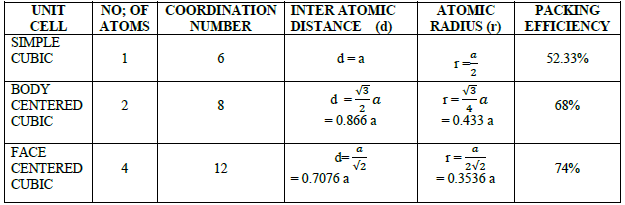## Tags:

Click for more Chemistry Study Material

## Latest NCERT & CBSE News

Read the latest news and announcements from NCERT and CBSE below. Important updates relating to your studies which will help you to keep yourself updated with latest happenings in school level education. Keep yourself updated with all latest news and also read articles from teachers which will help you to improve your studies, increase motivation level and promote faster learning

### BRICS International Online Mathematics Competition

BRICSMATH.COM is an annual International Online Competition in Mathematics, for students of classes I – XII of 07 BRICS countries (Brazil, Russia, India, China and South Africa, Indonesia and Vietnam). The competition is held online on the website www.bricsmath.com and...

### Tricks for Utilization of additional time introduced in CBSE Board Exams

Tricks for Proper Utilization of Add-On 15 Minutes introduced in CBSE Board Exams The intend of this article is to share the best suggestions and guidelines to utilize the extra 15 minutes provided for reading the question paper in CBSE Board Examination. These extra...

### Digital Marks Sheets Migration Certificates and Pass Certificates

CBSE pioneered in providing digital academic documents through its academic repository called “Parinam Manjusha” and DigiLocker.  As on now result data from 2004 till this year is available in the repository.  Approx. 12 crores digital academic documents are available...

### Celebration of Republic Day by CBSE

As you are aware, Republic Day is celebrated every year on 26th January to honour the Constitution and Republic of our country. Celebration of this occasion annually helps youth and children of our country to be aware of the significance of Indian Constitution, unity...

### How to Effectively Answer CBSE Board Examination Question Papers

The intend of this article is to share the best ways to answer the CBSE Board Examination. Usually most of the students tend to become nervous at the times of the board examination. In this article we will share the most effective ways to make the answers to the CBSE...

×